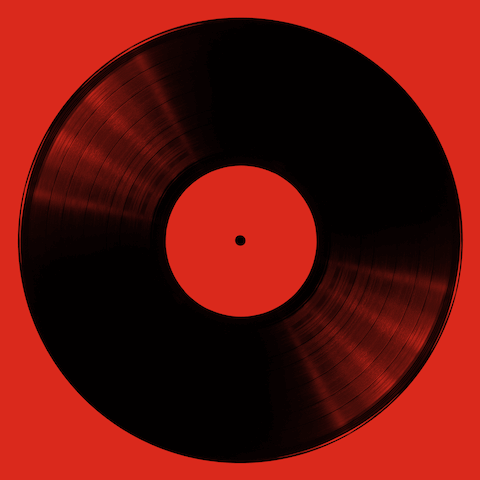• 12:12 am
• 12:12 am

12:12 am
• 12:12 am
• 12:12 am
• 12:12 am
• 12:12 am
• 12:12 am
• 12:12 am
• 12:12 am
• 12:12 am
• 12:12 am
• 12:12 am
• 12:12 am
• 12:12 am
• 12:12 am
• 12:12 am

• 12:12 am
• 12:12 am
• 12:12 am
• 12:12 am
• 12:12 am
• 12:12 am
• 12:12 am
• 12:12 am
• 12:12 am
• 12:12 am
• 12:12 am
• 12:12 am
• 12:12 am
• 12:12 am
• 12:12 am
• 12:12 am
• 12:12 am

• 12:12 am
• 12:12 am
• 12:12 am
• 12:12 am
• 12:12 am
• 12:12 am
• 12:12 am
• 12:12 am
• 12:12 am
• 12:12 am
• 12:12 am
• 12:12 am
• 12:12 am
• 12:12 am
• 12:12 am
• 12:12 am

• 12:12 am
• 12:12 am
• 12:12 am
• 12:12 am
• 12:12 am
• 12:12 am
• 12:12 am
• 12:12 am
• 12:12 am
• 12:12 am
• 12:12 am
• 12:12 am
• 12:12 am
• 12:12 am
• 12:12 am
• 12:12 am

• 12:12 am
• 12:12 am
• 12:12 am
• 12:12 am
• 12:12 am
• 12:12 am
• 12:12 am
• 12:12 am
• 12:12 am
• 12:12 am
• 12:12 am
• 12:12 am
• 12:12 am
• 12:12 am
• 12:12 am

• 12:12 am
• 12:12 am
• 12:12 am
• 12:12 am
• 12:12 am
• 12:12 am
• 12:12 am
• 12:12 am
• 12:12 am
• 12:12 am
• 12:12 am
• 12:12 am
• 12:12 am
• 12:12 am
• 12:12 am
• 12:12 am
• 12:12 am

• 12:12 am
• 12:12 am
• 12:12 am
• 12:12 am
• 12:12 am
• 12:12 am
• 12:12 am
• 12:12 am
• 12:12 am
• 12:12 am
• 12:12 am
• 12:12 am
• 12:12 am
• 12:12 am
• 12:12 am
• 12:12 am
• 12:12 am
• 12:12 am

• 12:12 am
• 12:12 am
• 12:12 am
• 12:12 am
• 12:12 am
• 12:12 am
• 12:12 am
• 12:12 am
• 12:12 am
• 12:12 am
• 12:12 am
• 12:12 am
• 12:12 am
• 12:12 am
• 12:12 am
• 12:12 am
• 12:12 am

• 12:12 am
• 12:12 am
• 12:12 am
• 12:12 am
• 12:12 am
• 12:12 am
• 12:12 am
• 12:12 am
• 12:12 am
• 12:12 am
• 12:12 am
• 12:12 am
• 12:12 am
• 12:12 am
• 12:12 am
• 12:12 am
• 12:12 am

• 12:12 am
• 12:12 am
• 12:12 am
• 12:12 am
• 12:12 am